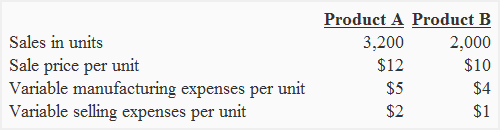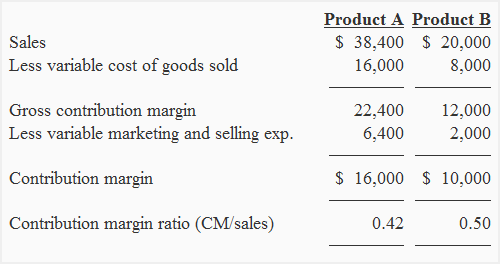Contribution margin ratio

Definition:

Contribution margin ratio (CM ratio) is the ratio of contribution margin to net sales. It tells what percentage of sales revenue is available to cover fixed cost and generate profit.

Formula:

Contribution margin ratio is calculated by dividing contribution margin figure by the net sales figure. The formula can be written as follows:The ratio is also shown in percentage form as follows:The numerator of the formula i.e., contribution margin can be calculated using simple contribution margin equation or by preparing a contribution margin income statement.

Examples:

Example 1

The total sales revenue of Black Stone Crushing Company was \$150,000 for the last year. The fixed and variable expenses data of the last year is given below:

Variable expenses:
Manufacturing: \$60,000
Marketing and administrative: \$30,000

Fixed:
Manufacturing: \$10,000
Marketing and administrative: \$8,000

Required: Calculate contribution margin ratio and also express it in percentage form.

Solution:

Contribution margin = \$150,000 – (\$60,000 + \$30,000)

= \$150,000 – \$90,000

= \$60,000

Contribution margin ratio = \$60,000/\$150,000

= 0.4

Contribution margin percentage = (\$60,000/\$150,000) × 100

= 40%

The contribution margin is 40% of net sales which means 40% of sales revenue is available to cover all fixed expenses and generate profit for the business.

Example 2:

The Monster company manufactures and sells two products. The data relating to sales and expenses for the last six months is given below:The total fixed expenses for the last six months were \$60,000. Out of these fixed expenses, 50% were manufacturing and remaining 50% were related to marketing and administrative activities. There were no beginning and ending inventories.

Required: Which product has the higher contribution margin ratio?

Solution:Product A’s contribution margin ratio is 0.42 or 42% where as product B’s contribution margin ratio is 0.5 or 50%. Product B is contributing more for covering fixed expenses and generating profit because its contribution margin ratio is higher than product A.

Show your love for us by sharing our contents.

4 Comments on Contribution margin ratio

1.Lammeck KAJUBI, PE, MBA

The contribution margin shows total sales revenue less variable costs of a profit center. It indicates ability of a profit center to control its variable costs and make a profit. Its importance is in enabling management to compare contribution margins of a multi-product setting, to decide which profit centers should remain in operation and which should be closed based on their individual contribution margins.

It is not always necessary or even possible to close production/ business in products of unfavourable contribution margins but to raise contribution margins of such products, key questions for any manager would be:

– how to raise sales
– how to lower variable costs and maintain production capability

2.Lissa

This is way better than a brick & mortar esthmlisabent.

3.wang zhang

very understandable

4.Ayaz Siddiqui

how to calculate cm ratio, if total fixed cost is given rather a sales revenue